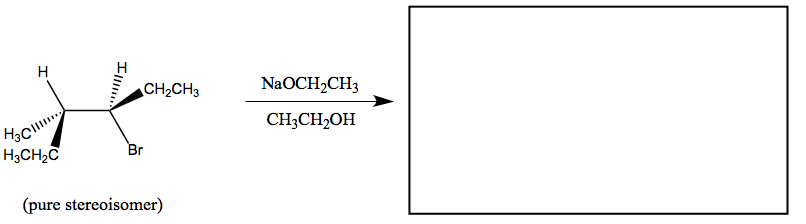# Problem: For the following reaction, provide a structural formula for the  major organic product(s). If no reaction occurs, write N.R. If the major product is a mix of stereoisomers, give a structural formula for each of the stereoisomers in the mixture. Also, write equal if equal amounts of stereoisomers are formed, or unequal if unequal amounts of stereoisomers are formed.

###### Problem Details

For the following reaction, provide a structural formula for the  major organic product(s). If no reaction occurs, write N.R. If the major product is a mix of stereoisomers, give a structural formula for each of the stereoisomers in the mixture. Also, write equal if equal amounts of stereoisomers are formed, or unequal if unequal amounts of stereoisomers are formed.What scientific concept do you need to know in order to solve this problem?

Our tutors have indicated that to solve this problem you will need to apply the Zaitsev Rule concept. You can view video lessons to learn Zaitsev Rule. Or if you need more Zaitsev Rule practice, you can also practice Zaitsev Rule practice problems.

What is the difficulty of this problem?

Our tutors rated the difficulty ofFor the following reaction, provide a structural formula for...as medium difficulty.

How long does this problem take to solve?

Our expert Organic tutor, Chris took 3 minutes and 33 seconds to solve this problem. You can follow their steps in the video explanation above.

What professor is this problem relevant for?

Based on our data, we think this problem is relevant for Professor Finzel's class at HOFSTRA.